# 3. A capacitor consists of two large metal disks of radius 2.5 meters placed parallel to...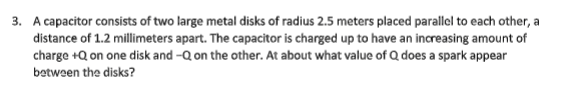3. A capacitor consists of two large metal disks of radius 2.5 meters placed parallel to each other, a distance of 1.2 millimeters apart. The capacitor is charged up to have an increasing amount of charge +Q on one disk and on the other. At about what value of Qdoes a spark appear between the disks?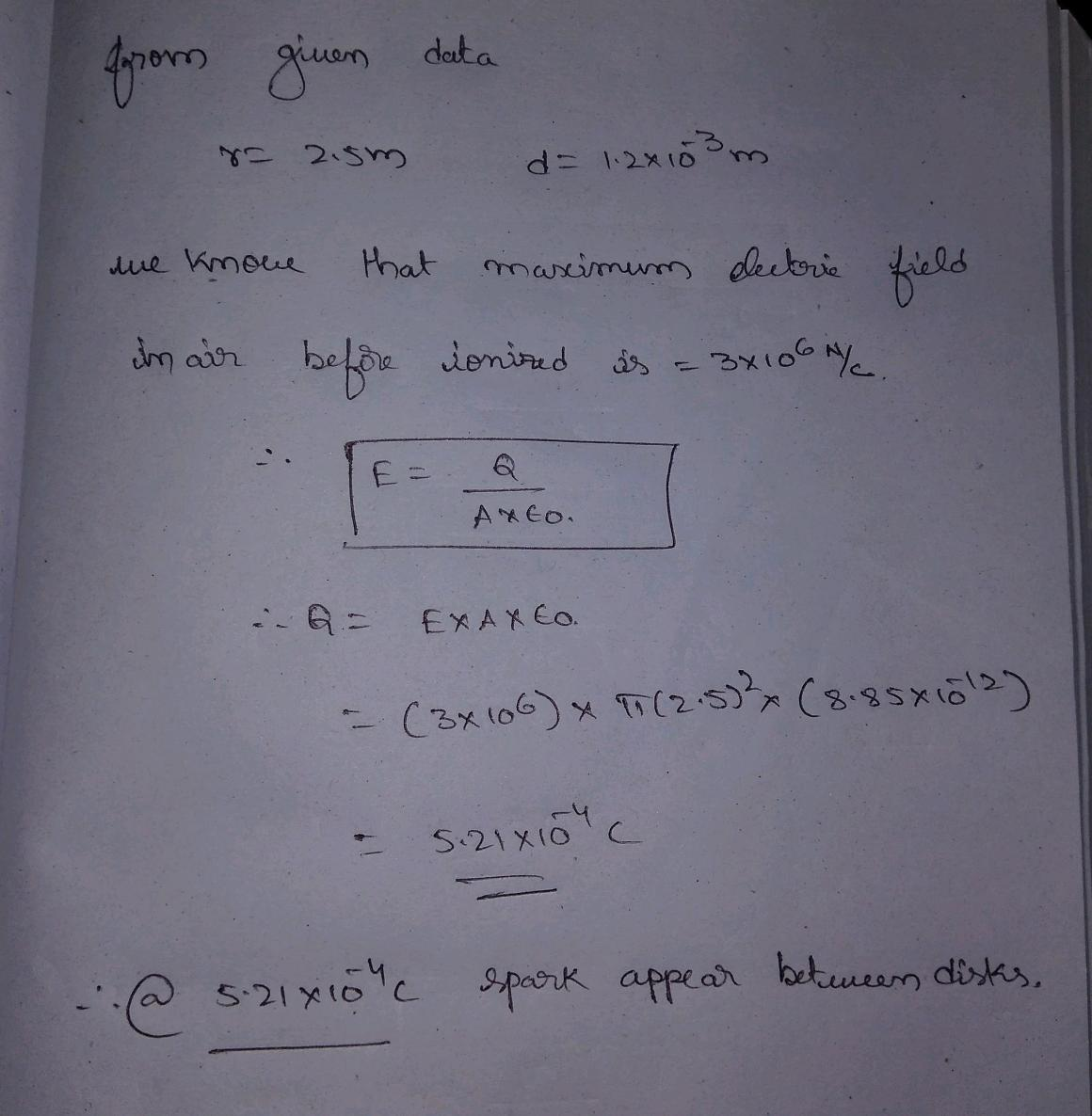#### Earn Coin

Coins can be redeemed for fabulous gifts.

Similar Homework Help Questions
• ### A capacitor consists of two charged disks of radius 5 m separated by a distance s...

A capacitor consists of two charged disks of radius 5 m separated by a distance s = 2 mm (see the figure). The magnitude of the charge on each disk is 58

• ### Two disks of diameter d are spaced a distance r apart to form a parallel-plate capacitor....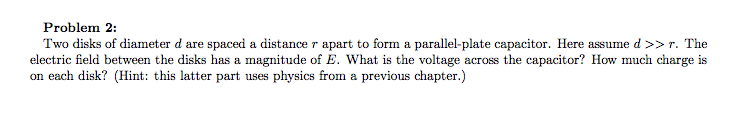Two disks of diameter d are spaced a distance r apart to form a parallel-plate capacitor. Here assume d >> r. The electric field between the disks has a magnitude of E. What is the voltage across the capacitor? How much charge is on each disk?

• ### A parallel plate capacitor consists of two circular metal plates of radius, R, separated by d...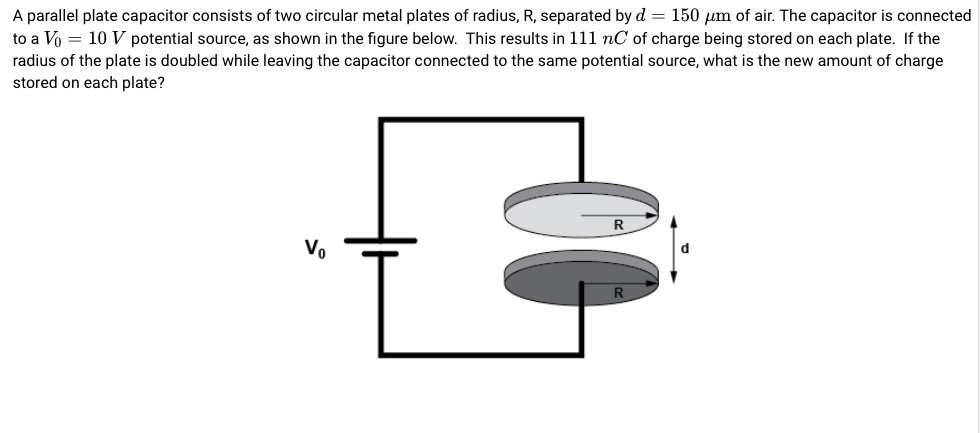A parallel plate capacitor consists of two circular metal plates of radius, R, separated by d = 150 μm of air. The capacitor is connected to a Vo 10 V potential source, as shown in the figure below. This results in 111 nC of charge being stored on each plate. If the radius of the plate is doubled while leaving the capacitor connected to the same potential source, what is the new amount of charge stored on each plate?

• ### A parallel plate capacitor consists of two circular metal plates of radius, R, separated by d...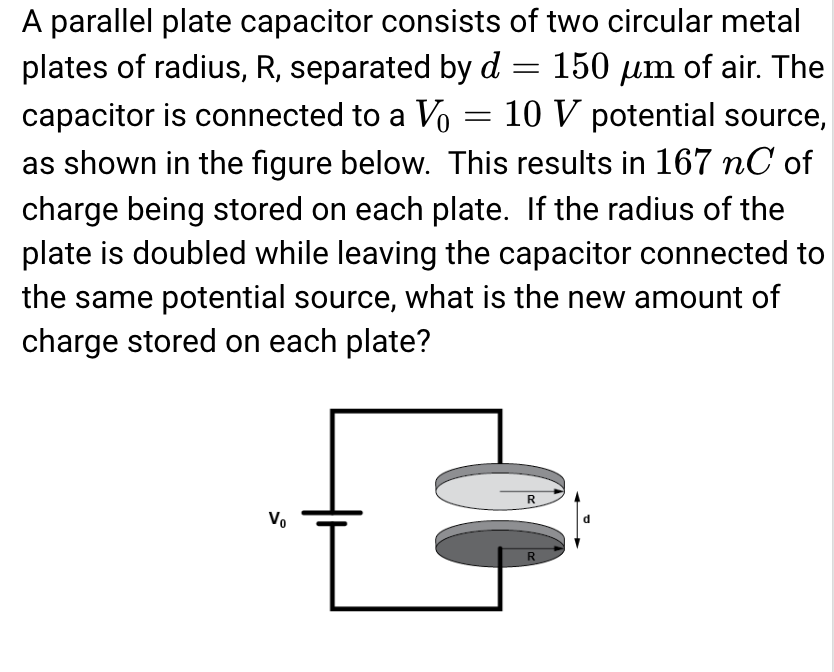A parallel plate capacitor consists of two circular metal plates of radius, R, separated by d -150 um of air. The capacitor is connected to a Vo- 10 V potential source, as shown in the figure below. This results in 167 nC of charge being stored on each plate. If the radius of the plate is doubled while leaving the capacitor connected to the same potential source, what is the new amount of charge stored on each plate?

• ### A parallel plate capacitor consists of two circular metal plates of radius, R, separated by d...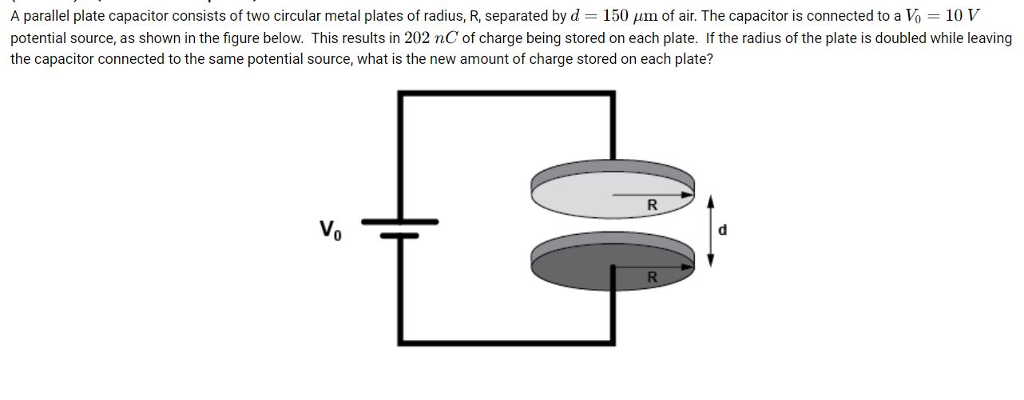A parallel plate capacitor consists of two circular metal plates of radius, R, separated by d 150 um of air. The capacitor is connected to a Vo 10 V potential source, as shown in the figure below. This results in 202 nC of charge being stored on each plate. If the radius of the plate is doubled while leaving the capacitor connected to the same potential source, what is the new amount of charge stored on each plate?

• ### Two 2.1-cm-diameter-disks spaced 1.6 mm apart form a parallel-plate capacitor. The electric field between the disks...

Two 2.1-cm-diameter-disks spaced 1.6 mm apart form a parallel-plate capacitor. The electric field between the disks is 4.8×105 V/m . What is the voltage across the capacitor? How much charge is on each disk?An electron is launched from the negative plate. It strikes the positive plate at a speed of 2.5×107 m/s . What was the electron's speed as it left the negative plate?

• ### Parallel Disk Capacitor

Two 1.90 cm-diameter disks are placed parallel to each other a distance 1.90 mm apart to form a capacitor. The electric field between the disks is 5.00×105 V/m.(Assume the field between the disks is constant.)A) What is the voltage (electric potential difference) between the two plates of the capacitor?B) How much charge is on each disk?C) An electron is launched from the negative plate. It strikes the positive plate at a speed of 2.40×107 m/s. What was the electron's speed...

• ### Parallel Disk Capacitor

Two 2.50 cm disks are placed parallel to each other a distance 2.00 mm apart to form a capacitor. The electric field between the disks is 4.90*10^5 V/m. (Assumethe field between the two disks is constant.)A) What is the voltage (electric potential difference) between the two plates of the capacitor? (V)B) How much charge is on each disk? (C)C) An electron is launched from the negative plate. It strikes the positive plate at a speed of 2.40*10^7 m/s. What was...

• ### 1.) A capacitor consists of two closely spaced metal conductors of large area, separated by a...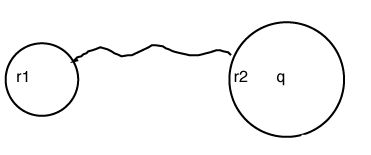1.) A capacitor consists of two closely spaced metal conductors of large area, separated by a thin insulating foil. It has an electrical capacity of 3800 uF and is charged to a potential difference of 75.0 V. Calculate the amount of energy stored in the capacitor. 2.)Calculate the charge on this capacitor when the electrical energy stored in the capacitor is 9.85 J. 3.)If the two plates of the capacitor have their separation increased by a factor of 4 while...

• ### A capacitor consists of two closely spaced metal conductors of large area, separated by a thin...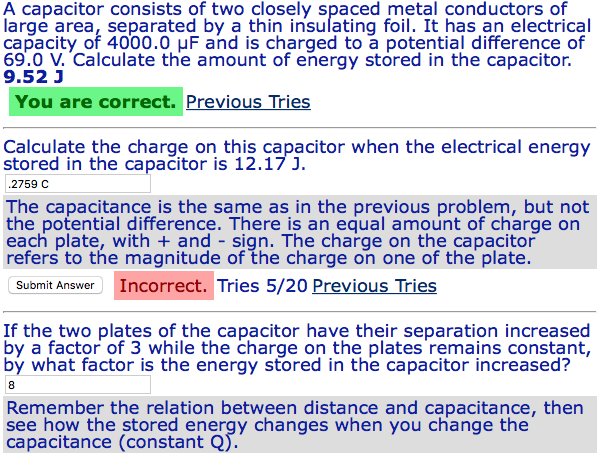A capacitor consists of two closely spaced metal conductors of large area, separated by a thin insulating foil. It has an electrical capacity of 4000.0 μF and is charged to a potential difference of 69.0 V. Calculate the amount of energy stored in the capacitor, 9.52 J You are correct. Previous Tries Calculate the charge on this capacitor when the electrical energy stored in the capacitor is 12.17 J. .2759 C The capacitance is the same as in the previous...